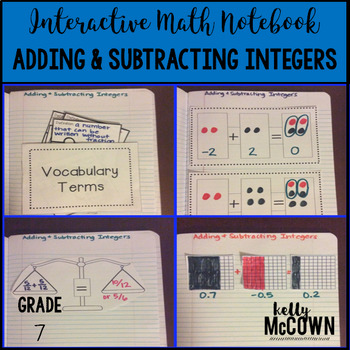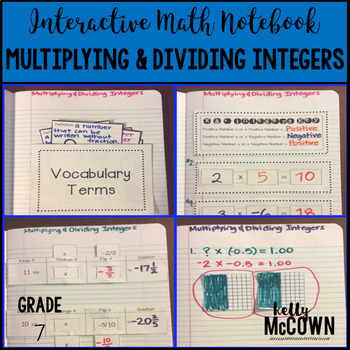## Monday, August 29, 2016

Let's take a look at teaching Integer Operations in 7th Grade! This is always a fun Math topic at the beginning of the school year to teach seventh graders. Students always come in with the prior knowledge of basic addition, subtraction, multiplication and division, but may never have heard of a dividing a negative number. Establishing the basic vocabulary of integers is key in understanding how to make connections of negative and positive integers.

Included in the Math Stations: Integers packet for seventh graders are some basic activities to practice making connections and developing concepts of Adding, Subtracting, Multiplying & Dividing Integers.This math station activity is intended to help students understand how to apply and extend previous understandings of addition and subtraction to rational numbers, describing situations in which opposite quantities combine, and showing that a number and its opposite have a sum of 0. Interpret sums of rational numbers by describing real world contexts. Students will also understand the additive inverse, apply properties of operations as strategies, and solve real-world problems with rational numbers.

Included are:

-6 different Integer stations to engage students

-Teacher facilitated activity for 60-90 minutes of classroom time

-Stations include:
1. Vocabulary
3. Practice of Adding & Subtracting Integers
5. Real World applications of Adding & Subtracting Integers
6. Word problems of Adding & Subtracting Integers

-Student Station Guide helps students record their answersThis math station activity is intended to help students understand how to apply and extend previous understandings of multiplication and division of fractions. Solve real-world and mathematical problems with four operations with rational numbers.

Included are:

-6 different Integer stations to engage students

-Teacher facilitated activity for 60-90 minutes of classroom time

-Stations include:
1. Vocabulary
3. Practice of Multiplying & Dividing Integers
4. Additional Practice of Multiplying & Dividing Integers
5. Real World applications of Integers
6. Word problems of Multiplying & Dividing Integers

-Student Station Guide helps students record their answers

Here are some other fun Integer activities for seventh graders to practice, apply, and get engaged in.Card Sort Activity & Assessment: Operations with Integers {Grade 7}I hope these activities help you to get some ideas about engaging your students in Operations with Integers. At the beginning of the year it's all getting your students ENGAGED in the learning process. Let them be able to enjoy MATH and have fun with the learning process!